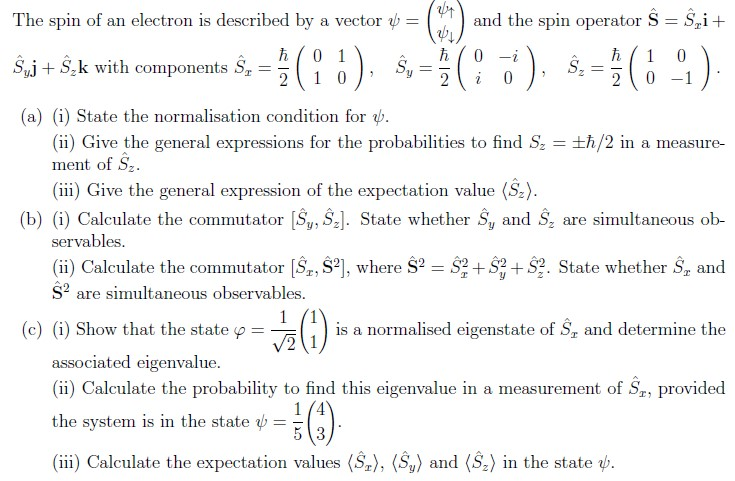QUESTION

# The spin of an electron is described by a vector [psi] = mat([psi_up],[psi_down]) and the spin operator S = Sxi+Syj+Szk with components Sx = (h/2)*mat([0,1],[1,0]), Sy = (h/2)*mat([0,-i],[i,0]), Sz = (h/2)*mat([1,0],[0,-1]). a)i) State the normalisation condition for [psi]. ii) Give the general expressions for the probabilities to find Sz =+-(h/2) in a measurement of Sz. iii) Give the general expression of the expectation value <Sz>. b)i) Calculate the commutator [Sy,Sz]. State whether Sy and Sz are simultaneous observables.  ii) Calculate the commutator [Sx,S2], where S2 = Sx2 + Sy2 + Sz2. State whether Sx and S2 are simultaneous observables.  c)i) Show that state [phi] = (1/sqrt(2))*mat(,) is a normalised eigenstate of Sx and determine the associated eigenvalue. ii) Calculate the probability to find this eigenvalue in a measurement of Sx, provided the system is in the state [phi] = (1/5)*mat(,). iii) Calculate the expectation values <Sx>, <Sy>, <Sz> in the state [psi]. Image with better formatted question is attached!